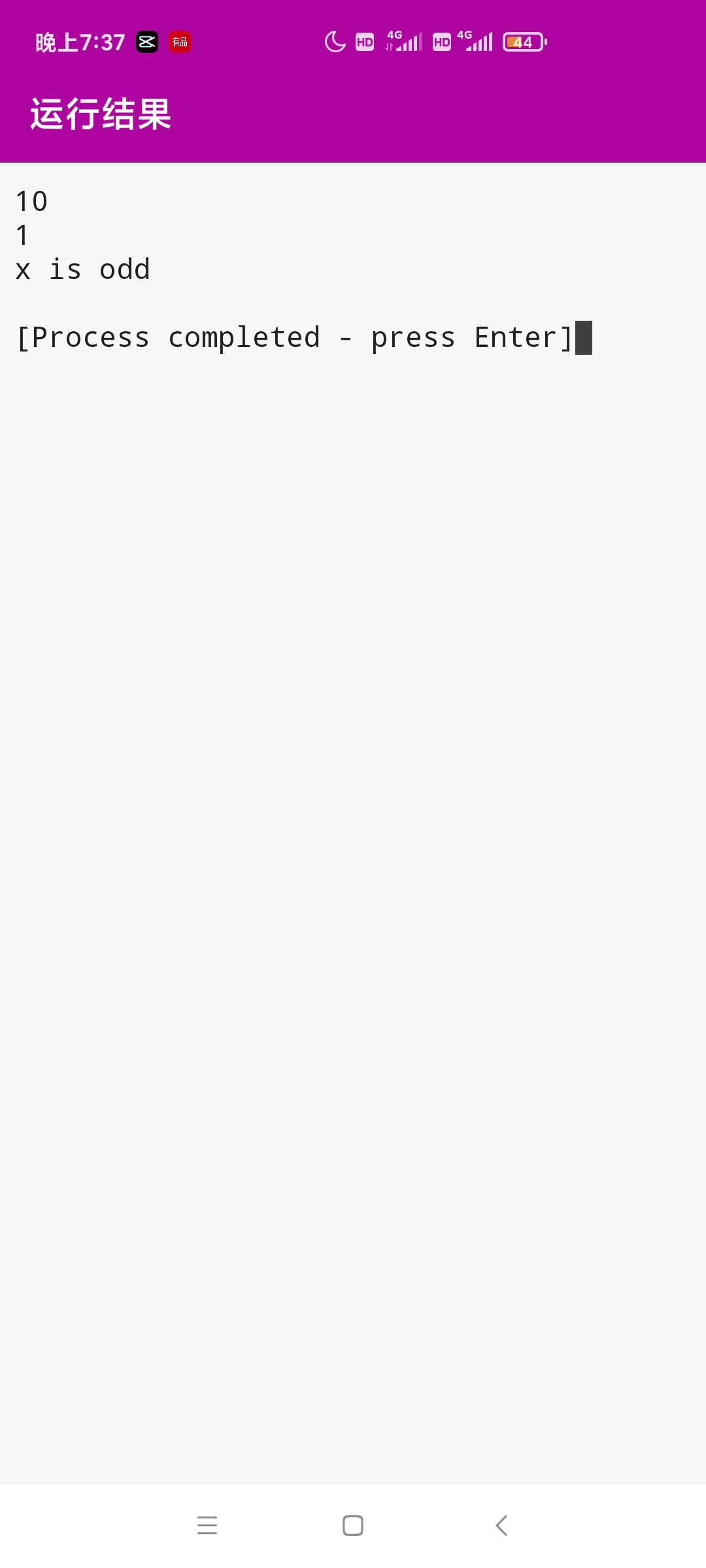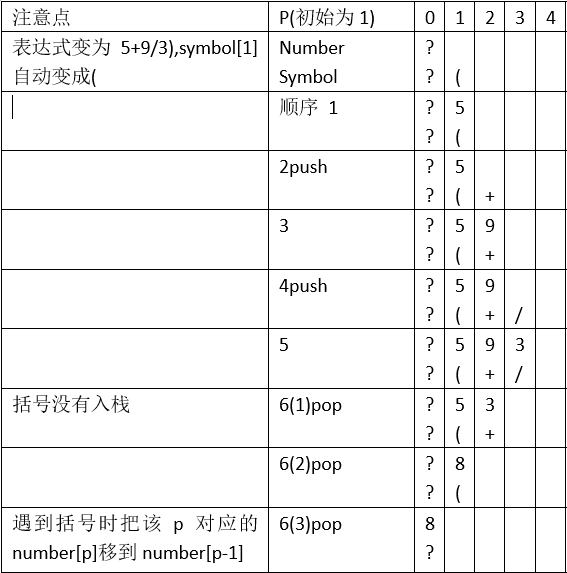• 一个能够计算表达式的java程序，支持算术运算、逻辑运算 安装jdk后双击可执行，使用解压软件打开后可查看源代码。Java 表达式运算
• 输入一个字符串表达式（如:1*(2+2^3)），返回表达式的解 。（不支持正负号）
• BeanShell java 表达式运算框架，及其方便快捷（附jar包，工具类，测试类）
• 带有（）的加减乘除运算，通过对运算符赋予优先级，词法分析，语法分析，及栈实现了运算
• 运算规则：首先计算表达式1的值， 若表达式1的值为真(非零) 则只计算表达式2，并将其结果作为整个表达式的值; 否则，只计算表达式3，并将其结果作为整个表达式的值。 #include<iostream> using namespace std;...

<表达式1>？<表达式2>：<表达式3>
运算规则：首先计算表达式1的值，
若表达式1的值为真(非零)
则只计算表达式2，并将其结果作为整个表达式的值;
否则，只计算表达式3，并将其结果作为整个表达式的值。

#include<iostream>
using namespace std;
int main()
{    int x=5,y=10,z;
z=x>y?x:y;
cout << z << endl;
z=x>0?1:-1;
cout<<z<<endl;
cout << (x%2==0?"x is even":"x is odd")<< endl;
return 0;}展开全文• 能进行以下运算：四则运算、乘方开方、三角函数、逻辑运算
• 超级函数表达式运算器既是方便实用的数字计算器，又是一个会列表达式就会运算的超级新颖的多功能公式化运算器。它包括算术计算、进制转换、逻辑处理、数值处理、字符处理、三角函数及推导函数处理等模块，可综合性的...
• 栈的应用 - 表达式运算

千次阅读 2018-11-05 10:42:35
中缀表达式：通常将运算符写在运算量之间，例如a+b，这种表示法称为中缀表示法。 后缀表达式：后缀表示法又称逆波兰表示法，它是波兰逻辑学家卢卡西维奇发明的一种表示表达式的方法。这种表示法把运算量写在前面，...

1 前缀、中缀、后缀表达式

前缀表达式：又称波兰表示法（Polish notation，或波兰记法），是一种逻辑、算术和代数表示方法，其特点是操作符置于操作数的前面，因此也称做前缀表示法。

中缀表达式：通常将运算符写在运算量之间，例如a+b，这种表示法称为中缀表示法。

后缀表达式：后缀表示法又称逆波兰表示法，它是波兰逻辑学家卢卡西维奇发明的一种表示表达式的方法。这种表示法把运算量写在前面，把运算符写在后面（后缀），例如a+b写作ab+，a+b*c写作abc*+，（a+b）*c写作ab+c*等等。

2 为什么要把中缀表达式转化为后缀，前缀？

计算机没法计算带有括号，以及区分优先级的表达式，或者说很难计算。使用后缀，前缀，消除了括号和优先级。

3 中缀表达式如何转化为后缀，前缀表达式？

转化为后缀：从左到右遍历中缀表达式，遇到操作数，输出，遇到操作符，当前操作符的优先级大于栈顶操作符优先级，进栈，否则，弹出栈顶优先级大于等于当前操作符的操作符，当前操作符进栈。

转化为前缀：从右到左遍历中缀表达式，遇到操作数，输出，遇到操作符，当前操作符的优先级大于等于栈顶操作符优先级，进栈，否则，弹出栈顶优先级大于当前操作符的操作符，当前操作符进栈。

4 中缀表达式转化为后缀具体实现（逆波兰式的实现）

在逆波兰式的实现中，最大的问题是如何改变运算符的先后顺序，把高优先级的运算符放在低优先级运算符的左侧。比如，有一个中缀表达式2 + 3 × 4，它所对应的逆波兰式应当是2 3 4 × +。

在我们做中缀表达式到逆波兰式转换的时候，必须在不改变操作数顺序的情况下，把高优先级的乘法操作符移动到低优先级的加法运算符的左侧。

很自然的，我们会想到堆栈。堆栈具有后进先出的特点。所以我们可以设计一个符号栈，用于临时存储一个中缀表达式中的全部运算符；设计一个RPN数组，用于存储逆波兰式输出。然后从左向右扫描一个中缀表达式，并循环执行以下步骤：

1. 如果扫描到一个运算数，则将其放到RPN数组的末尾
2. 如果扫描到一个运算符。首先比较该运算符和符号栈栈顶运算符的优先级。如果符号栈栈顶运算符的优先级比较低，则将新运算符压入符号栈（这样最后将符号出栈时，高优先级符号先出栈）。如果栈顶符号的优先级更高，就把栈顶符号弹出，并放入RPN数组末尾。然后继续比较新扫描到的运算符和栈顶运算符的优先级，直到找到一个优先级比自己低的栈顶元素或者到达堆栈栈底。
3. 如果表达式扫描结束。将栈中操作符顺序弹出放到RPN数组中。这样就得到了一个逆波兰表达式。

如下是一个逆波兰式生成过程的实例。

其中绿色部分代表每一步中发生变化的部分。其中特殊之处在于对括弧的处理，括弧的优先级高于所有操作符，但是括弧中所有的操作符优先级又高于括弧。所以当栈顶为括弧的时候，不会将括弧弹出，而是继续压栈。当弹出括弧的时候，也不会把括弧放入逆波兰式中。

原中缀表达式：3 + 4 × （5 - 2）/ 2

逆波兰表达式：3 4 5 2 - × 2 / +5 计算机如何计算后缀，前缀表达式？

计算后缀：从左到右遍历后缀表达式，遇到操作数，放进栈，遇到操作符，栈顶两个数出栈，进行运算，运算结果放进栈，直到读完后缀表达式。

计算前缀：从左到右遍历前缀表达式，遇到操作符，放进栈，遇到操作数，查看栈顶，栈顶为操作符，放进栈，栈顶为操作数，取出栈顶操作数和操作符，进行运算，运算后继续判断栈顶的情况。

6 计算机计算后缀表达式伪代码

while有输入符号
读入下一个符号
IF是一个操作数
入栈
ELSE IF是一个操作符
有一个先验的表格给出该操作符需要n个参数
IF堆栈中少于n个操作数
（错误） 用户没有输入足够的操作数
Else  n个操作数出栈
计算操作符。
将计算所得的值入栈
IF栈内只有一个值
这个值就是整个计算式的结果
ELSE多于一个值
（错误） 用户输入了多余的操作数

7 计算后缀表达式例子

中缀表达式 “5 + ((1 + 2) * 4) − 3” 写作后缀表达式  5 1 2 + 4 * + 3 −
下表给出了该逆波兰表达式从左至右求值的过程，堆栈栏给出了中间值，用于跟踪算法。

 输入 操作 堆栈 注释 5 入栈 5 1 入栈 5, 1 2 入栈 5, 1, 2 + 加法运算 5, 3 (1, 2)出栈；将结果（3）入栈 4 入栈 5, 3, 4 * 乘法运算 5, 12 (3, 4)出栈；将结果（12）入栈 + 加法运算 17 (5, 12)出栈；将结果 (17)入栈 3 入栈 17, 3 − 减法运算 14 (17, 3)出栈；将结果（14）入栈

计算完成时，栈内只有一个操作数，这就是表达式的结果：14

展开全文堆栈 后缀表达式 逆波兰表示法 计算后缀表达式
• 模拟表达式运算(带括号)

模拟表达式运算(加减乘除,负数,带括号)
要模拟表达式运算需要目标不同表达式之间的转换关系.
可以参考我的另一篇文章(中后缀表达式转换)
由于水平问题(自己写的总有bug,而且又长又繁琐),所以参考代码选取网上的优秀代码,
但网上的模拟表达式运算要么解释不清楚,要么代码不够完善.
所以我修改了一下网上代码,使其更简单,更完善.
这份代码用数组来表达堆栈.

运算数与运算符用同一个变量p表示栈顶下标.
因为双目运算符是两个元素进行运算,返回一个运算. n个运算数,则有n-1个双目运算符.(只包括双目运算符)
一个运算符对应它右边的运算数.(左括号用0对应,但0不参与运算,具体过程看下面代码)
最后返回1个最终结果.
按步骤分割程序:
(具体解释和细节都添加到注释中)
数据声明(全部采用全局变量):

int number;//储存运算数
char symbol;//储存运算符
char s;//储存字符串
int i=0;//用来遍历字符串
int p=1;//表达栈顶下标,初始化1是为了防止下标越界

入栈操作:

void push()//入栈
{
symbol[++p]=s[i];
//先增加p值然后将运算符置于栈顶.
}

出栈操作:(每输出一个就进行一次运算,就无需储存输出后的运算符)

void pop()//出栈,并顺带完成相应的运算
{//将number[p](栈顶)与number[p-1]进行运算,并将结果储存在number[p-1];
p--;//出栈后,栈顶下标-1;
//number[p]是新的栈顶元素
if(symbol[p+1]=='+')//已经弹出的运算符
{
number[p]+=number[p+1];//运算结果
}else if(symbol[p+1]=='-')
{
number[p]-=number[p+1];
}else if(symbol[p+1]=='*')
{
number[p]*=number[p+1];
}else if(symbol[p+1]=='/')
{
number[p]/=number[p+1];
}
}

返回运算符优先级:(可以加上其它优先级的运算符)

int cmp(char a)//返回运算符优先级
{
if(a=='*'||a=='/')
{
return 2;
}else if(a=='+'||a=='-')
{
return 1;
}else if(a=='(')//入栈的括号优先级最低
{
return 0;
}
return 0;
}

通过优先级比较判断出栈还是入栈:

int can()
{
if(cmp(s[i])<=cmp(symbol[p]))//当栈顶运算符优先级大于等于比较运算符时进行出栈
{
return 1;
}
return 0;
}

转换成数字:(可以用辅助字符数组记录数字,然后用atof转换,就可以处理浮点数)

int sum=0;
while(s[i]>='0'&&s[i]<='9')//转化数字
{
sum=sum*10+s[i++]-'0';
}
number[p]=sum;//设为栈顶运算数(因为下一个一定是运算符),初始化为0.

对运算符进行操作:

do{//一定进行一轮
if(s[i]==')')//遇到右括号,不入栈
{
while(symbol[p]!='(')//不是左括号则出栈
pop();
//栈顶为左括号时
number[--p]=number[p+1];//处理括号对应的0;
//此时symbol[p]为'(',因为'('对于的值是没有赋值的0,所以用栈顶的值代替括号对应的值.
}
else
{
if(s[i]=='-'&&s[i-1]=='*'||s[i-1]=='/')//处理负数 (单目运算符)
{number[p-1]*=-1;//改成负数
i++;
continue;
}
while(can())//一直出栈直到可以入栈
pop();
push();
}
i++;
}while(i<strlen(s)&&s[i-1]==')');//判断是否有多重括号

完全代码:

#include<iostream>
#include<cstdio>
#include<cstring>
using namespace std;
int number;//储存运算数
char symbol;//储存运算符
char s;//储存字符串
int i=0;//用来遍历字符串
int p=1;//表达栈顶下标,初始化1是为了防止下标越界
void push()//入栈
{
symbol[++p]=s[i];
//先增加p值然后将运算符置于栈顶.

}
void pop()//出栈,并顺带完成相应的运算
{//将number[p](栈顶)与number[p-1]进行运算,并将结果储存在number[p-1];
p--;//出栈后,栈顶下标-1;
//number[p]是新的栈顶元素
if(symbol[p+1]=='+')//已经弹出的运算符
{
number[p]+=number[p+1];//运算结果
}else if(symbol[p+1]=='-')
{
number[p]-=number[p+1];
}else if(symbol[p+1]=='*')
{
number[p]*=number[p+1];
}else if(symbol[p+1]=='/')
{
number[p]/=number[p+1];
}
}
int cmp(char a)//返回运算符优先级
{
if(a=='*'||a=='/')
{
return 2;
}else if(a=='+'||a=='-')
{
return 1;
}else if(a=='(')
{
return 0;
}
return 0;
}
int can()
{
if(cmp(s[i])<=cmp(symbol[p]))//当栈顶运算符优先级大于等于比较运算符时进行出栈
{
return 1;
}
return 0;
}
int main()
{
gets(s);
s[strlen(s)]=')';
symbol[p]='(';//symbol是括号,防止对空栈操作,同时对应第一个数
while(i<strlen(s))
{
while(s[i]=='(')//优先录入左括号
{
push();
i++;
}
int sum=0;
while(s[i]>='0'&&s[i]<='9')//转化数字
{
sum=sum*10+s[i++]-'0';
}
number[p]=sum;//设为栈顶运算数(因为下一个一定是运算符),初始化为0.
do{//一定进行一轮
if(s[i]==')')//遇到右括号,不入栈
{
while(symbol[p]!='(')//不是左括号则出栈
pop();
//栈顶为左括号时
number[--p]=number[p+1];//处理括号对应的0;
//此时symbol[p]为'(',因为'('对于的值是没有赋值的0,所以用栈顶的值代替括号对应的值.
}
else
{
if(s[i]=='-'&&s[i-1]=='*'||s[i-1]=='/')//处理负数 (单目运算符)
{number[p-1]*=-1;//改成负数
i++;
continue;
}
while(can())//一直出栈直到可以入栈
pop();
push();
}
i++;
}while(i<strlen(s)&&s[i-1]==')');//判断是否有多重括号
}
cout<<number<<endl;
return 0;
}

注意点:
1.开头就自动在首尾添上括号
2.先p++才入栈,同个p,symbo先被赋值
3.遇到左括号则将对应数值"左移".
4.number是最终结果.
该代码运行流程:
案例:5+9/3这段代码对于初学者来说可能很复杂,可以多调试几遍或画图来加深理解.
由于水平问题,文章中可能存在不足和错误,欢迎评论指出.

展开全文表达式运算
• 会写表达式，就可以计算的软件，很好很强大，安装后打开若提示缺少MSVBVM50，则只需要将其中的解压拷贝到c盘windows system32下即可。
• C语言实现栈实现表达式运算的应用，很好用，有需要的来下载。
• [Matlab]函数表达式运算

千次阅读 2018-11-21 20:41:16
MATLAB对函数表达式进行求和 有时候要进行函数表达式的计算 syms m; %m是符号，声明符号 f1=exp(-im*1); f2=exp(-im*2); f=f1+f2;%可以以符号的形式存储下来 %计算% subs(f,'m',1);%将f中的m替换为1 总结一下，...

MATLAB对函数表达式进行求和
有时候要进行函数表达式的计算

syms m; %m是符号，声明符号
f1=exp(-im*1);
f2=exp(-im*2);
f=f1+f2;%可以以符号的形式存储下来

%计算%
subs(f,'m',1);%将f中的m替换为1，最好用单引号把变量括起来

总结一下，syms用于声明符号，subs用于将符号替换为数字，进行计算。
syms了以后，还有很多函数用于求导积分什么的。
详情可:

help syms;
help subs;

p.s
记录一个现象，如果subs(f,m,1)，不加’’，对exp函数会无法将m替换。

展开全文matlab
• java 用户输入加减乘除表达式运算,用户输入解析部分很好用
• Problem B: 只有一个二元运算符的表达式运算 Time Limit: 1 Sec Memory Limit: 2 MB Submit: 12734 Solved: 5441 [Submit][Status][Web Board] Description 编程序读入并计算只有一个二元运算符的表达式的值。...
• java 与 script 进行交互的 实现源码，逻辑表达式 判断表达式 运算 等，含有测试方法，需要javax.script的jar包
• 曾经有个需求，要把一段字符串表达式作为C#的一段语句来执行。或者直接在sql中存储上不同的含参数的工时如180+20*（n-4)。查出来之后带入参数计算。...然后去运算（即自己写一个类和函数）2.采用T-sql脚本计算。
• 逻辑表达式和关系表达式 0表示假，1表示真，任何非0的数为真 逻辑表达式是指运算符为或||、与&amp;&amp;、非！的表达式。返回值为0或1，0表示false，非0表示true. 例如!0返回1，0&amp;&amp;1返回0...
• 1 - 2 * ((60-30 +(-40.0/5) * (9-2*5/3 + 7 /3*99/4*2998 +10 * 568/14 )) - (-4*3)/ (16-3*2)))通过Python实现，自动判断括号以及加减乘除的运算优先级，得出运算结果同时允许用户自己输入表达式（限+-*/()），...Python 正则表达式 计算器
• 用栈实现表达式运算

千次阅读 2015-03-18 16:53:26
（1）建立两个栈，一个用来存储操作数，另一个用来存储运算符,开始时在运算符栈中先压入‘/0’，一个表达式的结束符。 （2）然后从左至右依次读取表达式中的各个符号（操作数或者运算符）； （3）如果读到的是操作...C++ 数据结构
• 一个Delphi适用的数学表达式运算单元..rarDelphi
•表达式运算
• 定义函数表达式 f_1 = L*(tan(alpha_t+theta))/k_t; f_2 = L*(tan(alpha_r+theta))/k_r; f_3 = L*(tan(alpha_t))/k_t; f_4= L*tan(alpha_r) / k_r; f = (f_1-f_2) - (f_3 - f_4); 表达式化简以及正常显示 ...
• MATLAB符号表达式运算

万次阅读 2015-04-27 15:41:29
一旦创建了一个符号表达式，或许想以某些方式改变它；也许希望提取表达式的一部分，合并两个表达式或求得表达的数值。有许多符号工具可以帮助完成这些任务。 　所有符号函数(很少特殊例外的情况，讨论于后)作用到...matlab
•   最近在学安卓的相关知识，第一个demo做了一个简易计算器，功能仿手机上自带的计算器，没有加括号，简单的四则运算，支持长表达式运算。此篇重点讲解计算器的运算逻辑，如何处理长表达式运算。   虽然，此次的...Android 计算器 表达式运算 后缀表达式 中缀表达式
• 后缀表达式是计算机容易运算表达式,运算符在运算数后面,从左到右进行运算,无需考虑优先级,运算呈线性结构. 先举个简单的转换例子 2+9/3-5 (前缀)-> 2 9 3 / + 5 - (后缀) 先进行乘除再进行加减 运算规律,.....表达式运算 后缀表达式 中缀表达式
• 1：printf中的逗号表达式运算规律：printf中如果有俩个或俩个以上（逗号）表达式，那么其运算规则是从有向左边开始运算。(这与不在printf的逗号表达式不一样是不一样的） 并且表达式会影响下面语句的值。 2：逗号...c语言 算法 排序算法
• C实现的带括号表达式运算 遵守四则运算的优先级。
• 运用MFC 编写的表达式运算 采用计算器形式实现表达式运算...